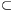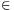Mathematical and Physical Journal
for High Schools
Issued by the MATFUND Foundation
 Already signed up? New to KöMaL?

#Problem A. 573. (November 2012)

A. 573. Let D={0,1,2,...,9} be the set of decimal digits, and let RD×D be a set of ordered pairs of digits. An infinite sequence (a1,a2,a3,...) of digits is said to be compatible with R if (aj,aj+1)R for all positive integer j. Determine the smallest positive integer K with the property that if an arbitrary set RD×D is compatible with at least K distinct digit sequences then R is compatible with infinitely many digit sequences.

Based on the 5th problem of CIIM 2012, Guanajuato, Mexico

(5 pont)

Deadline expired on December 10, 2012.

### Statistics:

 15 students sent a solution. 5 points: Ágoston Péter, Fehér Zsombor, Janzer Olivér, Maga Balázs, Omer Cerrahoglu, Vályi András, Williams Kada, Zilahi Tamás. 4 points: Herczeg József, Ioan Laurentiu Ploscaru. 2 points: 2 students. 1 point: 1 student. 0 point: 2 students.

Problems in Mathematics of KöMaL, November 2012